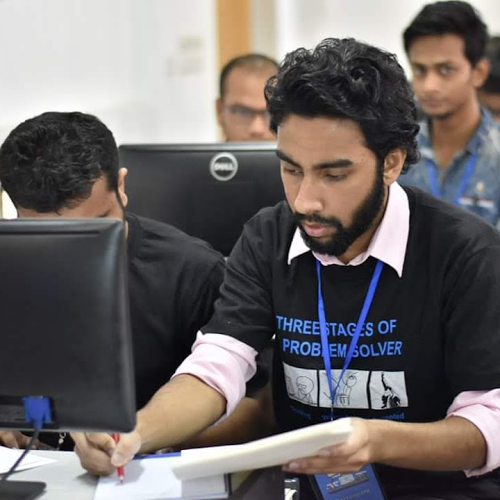# El Disinfecto

IR-Beacon Guided Autonomous Disinfection Robot Using UVC and Junkyard Material for Cheap & Fast Production

IntermediateFull instructions providedOver 3 days748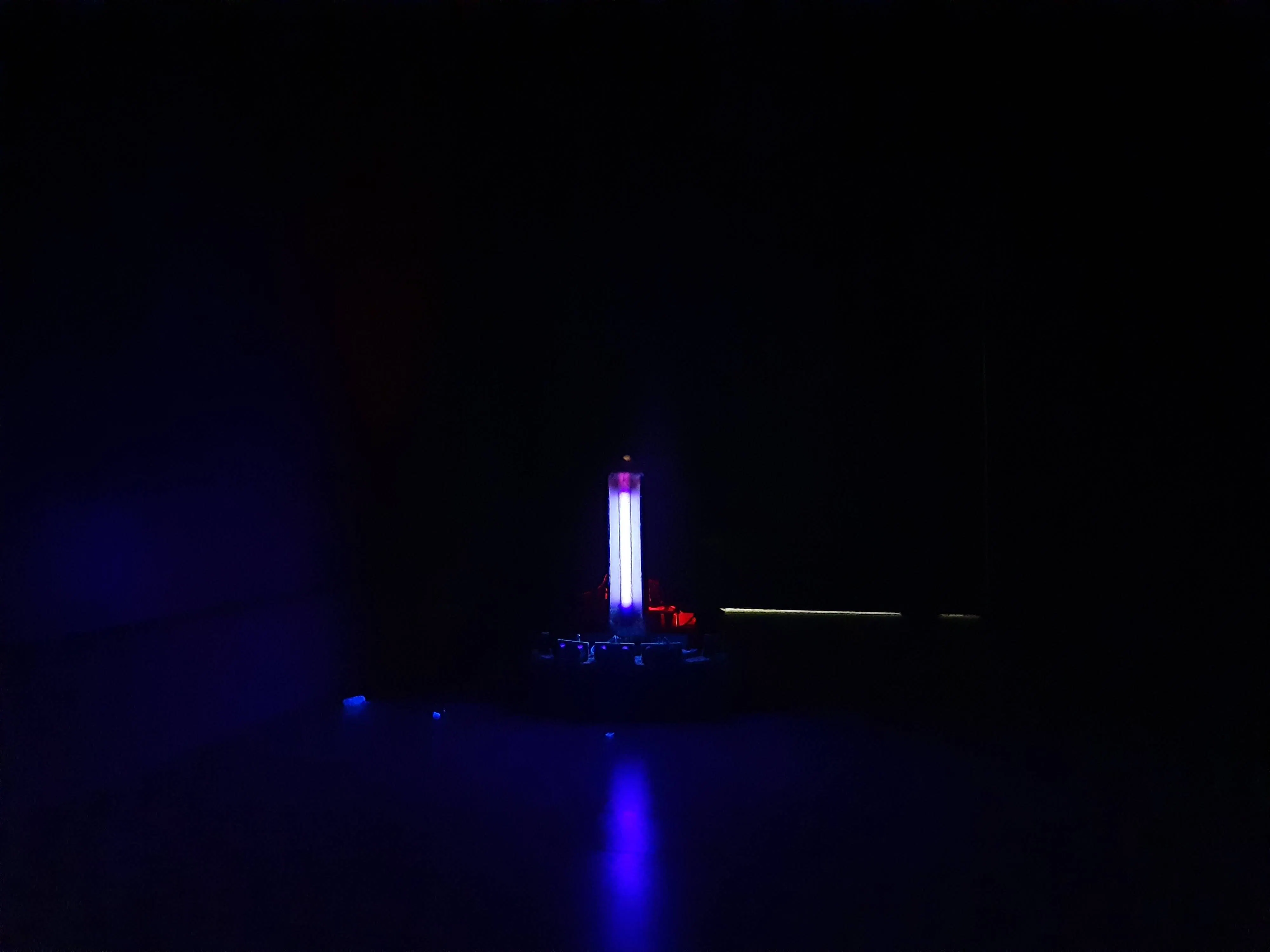## Things used in this project

### Hardware components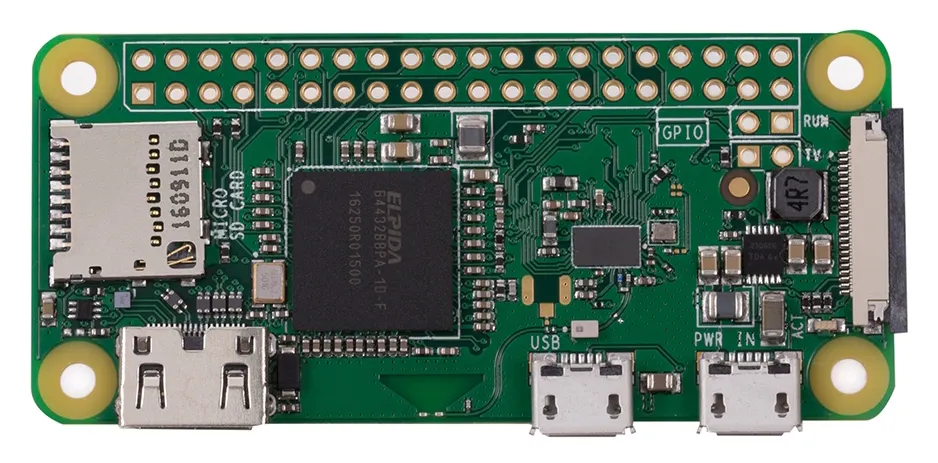Raspberry Pi Zero Wireless
×1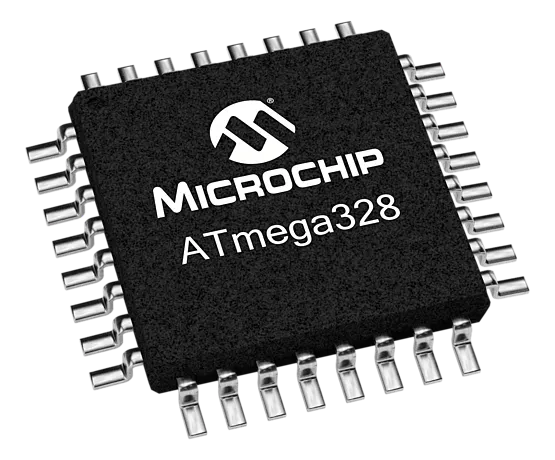Microchip Technology ATmega328
×1
 3300mAh LiPo battery
×1
 1100mAh LiPo battery
×1
 4 W UV lamp
×1
×2Ultrasonic Sensor - HC-SR04 (Generic)
×5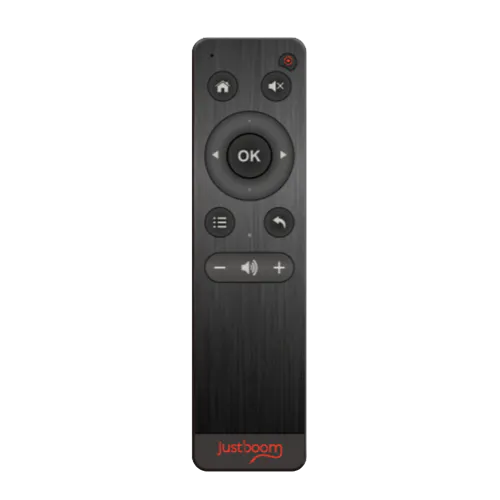JustBoom IR Remote
×1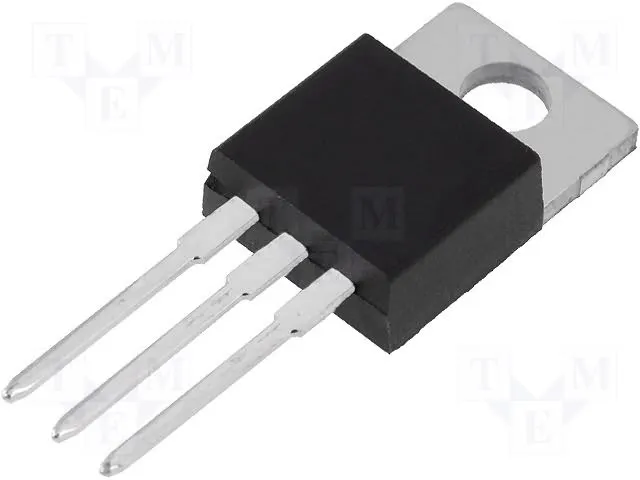Linear Regulator (7805)
×3
 Wifi Adapter Though we had integrated wifi module in our raspberry pi but we used an external one for better connectivity.
×1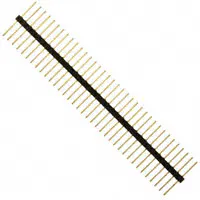Male-Header 36 Position 1 Row- Long (0.1")
×2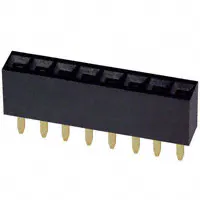Female Header 8 Position 1 Row (0.1")
×7
 Vero board
×1Jumper wires (generic)
×1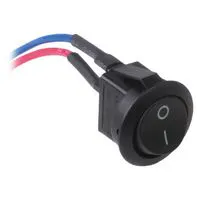Rocker Switch, SPST
×1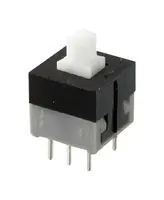Pushbutton Switch, Push-Pull
×1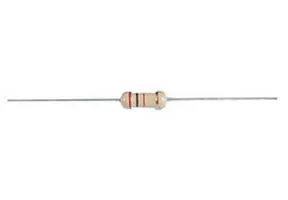Resistor 220 ohm
×1Through Hole Resistor, 82 ohm
×1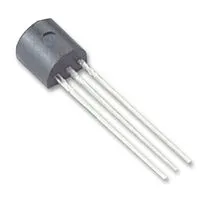General Purpose Transistor NPN
×1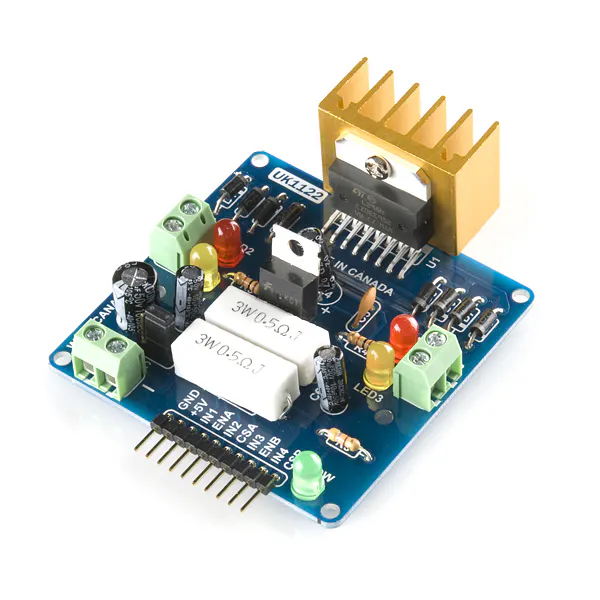SparkFun Dual H-Bridge motor drivers L298
×1
 Dc motor with wheels
×1LED (generic)
×1
 Ball caster
×1

### Software apps and online servicesRaspberry Pi RaspbianArduino IDE
 LiveDroid: Wireless WebCam

### Hand tools and fabrication machines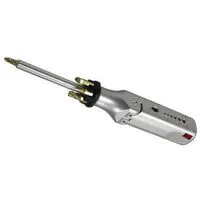Multitool, ScrewdriverSoldering iron (generic)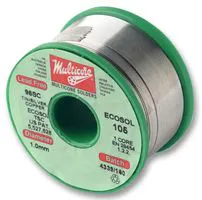Solder Flux, Soldering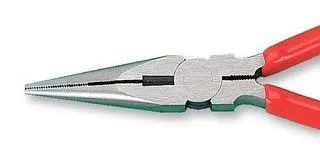Plier, Long NoseHot glue gun (generic)Drill / Driver, Cordless
 Angle grinder

## Custom parts and enclosures

### Chassis Design

This AutoCAD design file includes all the measurements in our design procedure.

## Schematics

### Power and Brain

This circuit diagram is the heart of the system. It powers Raspberry pi, Micro-controller ATMega328p, l298N Motor driver, all the sensors including ultrasonic sensor and IR receiver.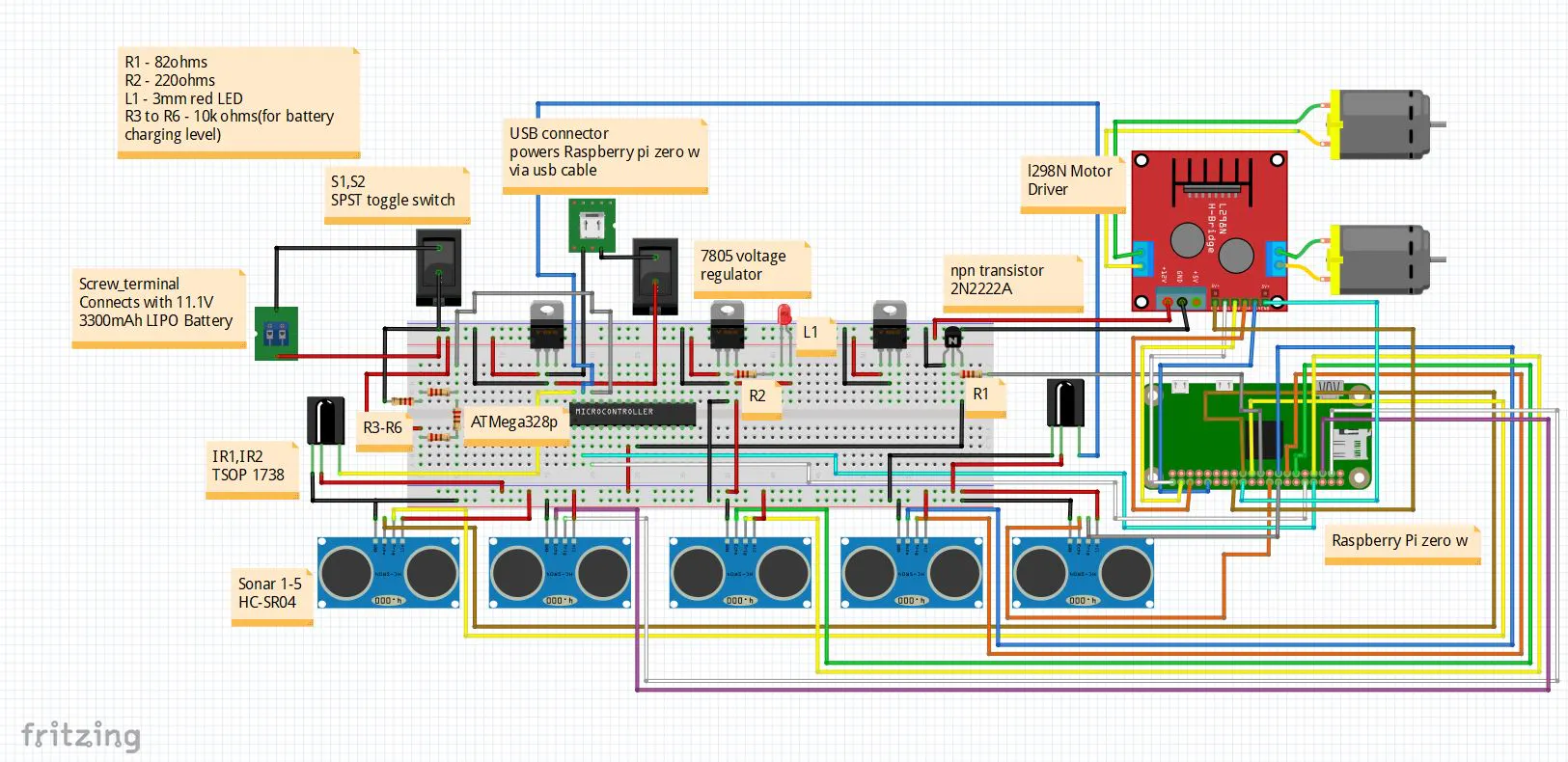## Code

Python
As we have used five sonar sensors. And all of them are integrated with raspberry pi. This code helps to get distance using all those five sensors. and the read() function will be used from another python script.
```import RPi.GPIO as GPIO
import time

GPIO.setmode(GPIO.BCM)
GPIO_TRIGGER = [2,4,27,9,23]
GPIO_ECHO = [3,17,22,11,24]

left=0
middle=0
right=0
right_most=0
left_most=0

for i in range(5):
GPIO.setup(GPIO_TRIGGER[i], GPIO.OUT)
GPIO.setup(GPIO_ECHO[i], GPIO.IN)

def distance(i):

GPIO.output(GPIO_TRIGGER[i], False)

time.sleep(0.00002)
GPIO.output(GPIO_TRIGGER[i], True)

time.sleep(0.0001)
GPIO.output(GPIO_TRIGGER[i], False)
time.sleep(0.00002)

StartTime = time.time()
StopTime = time.time()

while GPIO.input(GPIO_ECHO[i]) == 0:
StartTime = time.time()

while GPIO.input(GPIO_ECHO[i]) == 1:
StopTime = time.time()

TimeElapsed=StopTime - StartTime

distance=(TimeElapsed * 34300) / 2

return distance

global left
global right
global middle
global left_most
global right_most

left = distance(0)
time.sleep(0.3)
middle=distance(1)
time.sleep(0.3)
right=distance(2)
time.sleep(0.3)
left_most=distance(3)
time.sleep(0.3)
right_most=distance(4)
time.sleep(0.3)

```

### Motor Drive

Python
We have used this python script to run our motors.
```import RPi.GPIO as GPIO
import time

pinA=21
pinB=20
pinC=16
pinD=12

en1=7
en2=8

GPIO.setmode(GPIO.BCM)
GPIO.setup(pinA,GPIO.OUT)
GPIO.setup(pinB,GPIO.OUT)
GPIO.setup(pinC,GPIO.OUT)
GPIO.setup(pinD,GPIO.OUT)

GPIO.setup(en1,GPIO.OUT)
GPIO.setup(en2,GPIO.OUT)
duty1=38
duty2=38
p1=GPIO.PWM(en1, 500)
p2=GPIO.PWM(en2, 500)
p1.start(duty1)
p2.start(duty2)

def clean():
GPIO.cleanup()

def fwd():
print("Forward called..")
GPIO.output(pinA, GPIO.LOW)
GPIO.output(pinB, GPIO.HIGH)
GPIO.output(pinC, GPIO.HIGH)
GPIO.output(pinD, GPIO.LOW)

def bck():
print("Backward called..")
GPIO.output(pinA, GPIO.HIGH)
GPIO.output(pinB, GPIO.LOW)
GPIO.output(pinC, GPIO.LOW)
GPIO.output(pinD, GPIO.HIGH)

def left():
print("Left called..")
GPIO.output(pinA, GPIO.LOW)
GPIO.output(pinB, GPIO.HIGH)

def right():
print("Right called..")
GPIO.output(pinC, GPIO.HIGH)
GPIO.output(pinD, GPIO.LOW)

def stop():
print("Stop called..")
GPIO.output(pinA, GPIO.LOW)
GPIO.output(pinB, GPIO.LOW)
GPIO.output(pinC, GPIO.LOW)
GPIO.output(pinD, GPIO.LOW)

def drive(move):
try:
if(move=='w'):
fwd()
#time.sleep(0.2)
elif(move=='s'):
bck()
elif(move=='a'):
left()
elif(move=='d'):
right()
elif(move=='p'):
stop()

except KeyboardInterrupt:
GPIO.cleanup()
```

Python
We have set up serial communication between micro-controller ATMega328p and raspberry pi zero w. This python script helps to read the data which was sent from the micro-controller.
```import time
import serial

ser = serial.Serial(
port='/dev/ttyS0',
baudrate = 9600,
parity=serial.PARITY_NONE,
stopbits=serial.STOPBITS_ONE,
bytesize=serial.EIGHTBITS,
timeout=1
)
counter=0

return x

```

Python
We have used this python script to guide our robot, drive the motors by listening the serial data which was sent from ATMega328p.
```import serial_read
import time
import motor
import RPi.GPIO as GPIO
import sonar
import RPi.GPIO as GPIO

enable = 10

GPIO.setmode(GPIO.BCM)
GPIO.setup(enable,GPIO.OUT)

def getMillis():
return int(round(time.time() * 1000))

GPIO.output(enable, GPIO.HIGH)

try:

while(True):
#print(type(data))
motor.drive("p")
startTime = getMillis()
while(getMillis() - startTime <= 3000):
motor.drive("p")
time.sleep(0.1)
if(sonar.middle<2):
print("both sonar")
motor.drive("s")
time.sleep(0.35)
motor.drive("p")
time.sleep(0.01)
motor.drive("a")
time.sleep(0.08)

elif(sonar.left<=10):
motor.drive("p")
print("sonar left")
motor.drive("s")
time.sleep(0.35)
motor.drive("p")
time.sleep(0.01)
motor.drive("a")
time.sleep(0.08)

elif(sonar.right<=10):
motor.drive("p")
print("sonar right")
motor.drive("s")
time.sleep(0.35)
motor.drive("p")
time.sleep(0.01)
motor.drive("d")
time.sleep(0.08)

elif(sonar.left_most<=10):
motor.drive("p")
print("sonar left most")
motor.drive("s")
time.sleep(0.3)
motor.drive("p")
time.sleep(0.01)
motor.drive("a")
time.sleep(0.08)

elif(sonar.right_most<=10):
motor.drive("p")
print("sonar right most")
motor.drive("s")
time.sleep(0.3)
motor.drive("p")
time.sleep(0.01)
motor.drive("d")
time.sleep(0.08)

GPIO.output(enable, GPIO.LOW)
GPIO.output(enable, GPIO.HIGH)
startTime = getMillis()
while(getMillis() - startTime <= 1000):
stringData = data.rstrip()
print(stringData)

if(stringData=="forward"):
motor.drive("w")
time.sleep(0.1)
#motor.drive("p")
#print(data)
elif(stringData=="left"):
motor.drive("a")
time.sleep(0.1)
motor.drive("p")
#print(data)
elif(stringData=="right"):
motor.drive("d")
time.sleep(0.1)
motor.drive("p")
#print(data)
elif(stringData=="stop"):
motor.drive("p")
#motor.drive(move)
#print(move)

except KeyboardInterrupt:

GPIO.cleanup()
print("Terminated")
```

### Manual Drive using Firebase Database

Python
In our manual drive section. we have used an integrated web app and Firebase database to store the change of the key values. By these values raspberry pi gets to know which action to take. decides between forward,left,right.
```from firebase import firebase
import RPi.GPIO as GPIO
import time
import motor

firebase=firebase.FirebaseApplication("https://acr-project-8f9b6.firebaseio.com/",None)
pushed=firebase.get('/Test','')

try:
while 1:
pushed=firebase.get('/Test','')
if(pushed['up_btn']):
motor.drive("w")
elif(pushed['down_btn']):
motor.drive("s")
elif(pushed['left_btn']):
motor.drive("a")
elif(pushed['right_btn']):
motor.drive("d")
else:
motor.drive("p")

except KeyboardInterrupt:
GPIO.cleanup()
```

### Battery Charging Level Detection

Arduino
We need to continuously measure the battery percentage. This Arduino code helps to know the battery voltage by applying simple voltage divider rule. As we have implemented serial communication between raspberry pi and micro-controller, so the raspberry pi can decide what to do with battery level.
```int analogInput = A0;
float vout = 0.0;
float vin = 0.0;
float R1 = 30000.0;
float R2 = 10000.0;
int value = 0;

void setup(){
Serial.begin(9600);
}

void loop(){
vout = (value * 5) / 1024.0;
vin = vout / (R2/(R1+R2));
Serial.println(vin);
delay(500);
}
```

### IR Receiver Data Process and Execution

Arduino
As we have used IR receiver, which sends analogue value. So, the analogue value process and the whole decision is made on this Arduino code. Raspberry pi listens to the serial port and when gets string like:"forward", it then tells the motor to act accordingly.
```int analogInputRightIr = A5;
unsigned long int waitTime=50;
int left_delay=25;
int right_delay=25;
int left_count=0;
int right_count=0;
int runTime = 100;

void setup() {
Serial.begin(9600);
}

void loop() {

unsigned long int startTime=millis();
while(millis()-startTime<=100){
if(rightIrGotHit())
right_count++;
delayMicroseconds(10);

if(leftIrGotHit())
left_count++;
delayMicroseconds(10);
}

if(abs(left_count-right_count)==2 && (left_count-right_count!=0)){
left_count=0;
right_count=0;
forward();
}
if(left_count>right_count){
left_count=0;
right_count=0;
left();
}
if(left_count<right_count){
left_count=0;
right_count=0;
right();
}

stopp();

}

boolean leftIrGotHit(){

delayMicroseconds(left_delay);
int pulseCount=0;
unsigned long int startTime = millis();
unsigned long int currentTime = millis();
while(abs(startTime-currentTime)<=waitTime){
if(abs(PreValueLeftIr - PostValueLeftIr)>30){
pulseCount+=1;
}
delayMicroseconds(left_delay);
currentTime = millis();
if(pulseCount>=3){
//        Serial.println("Go Left");
return true;
}
}
return false;
}

boolean rightIrGotHit(){

delayMicroseconds(right_delay);
int pulseCount=0;
unsigned long int startTime = millis();
unsigned long int currentTime = millis();
while(abs(startTime-currentTime)<=waitTime){
if(abs(PreValueRightIr - PostValueRightIr)>30){
pulseCount+=1;
}
delayMicroseconds(right_delay);
currentTime = millis();
if(pulseCount>=3){
//        Serial.println("Go Right");
return true;
}
}
return false;

}

void forward(){

int countForward = 2;
int nextSetupTime = 0;
unsigned long int startTime=millis();
while(millis()-startTime<=runTime){

Serial.println("forward");

if(rightIrGotHit())
right_count++;

if(leftIrGotHit())
left_count++;

if(abs(left_count-right_count)==2){
nextSetupTime = 1*countForward++ ;
}
}
runTime -= nextSetupTime;
}

void left(){

Serial.println("left");
delayMicroseconds(10);

}

void right(){

Serial.println("right");
delayMicroseconds(10);

}

void stopp(){

Serial.println("stop");

}

```

### Manual Drive with Live feedback

Web based app to stream live feedback sent from the livedroid android application running on a mobile device which is mounted on top of the robot. It has also integrated controls for manual driving.

## Credits

### Rahul Mohoto

0 projects • 0 followers

### Tasfik Rahman

0 projects • 0 followers

### Akib Uz Zaman

0 projects • 0 followers